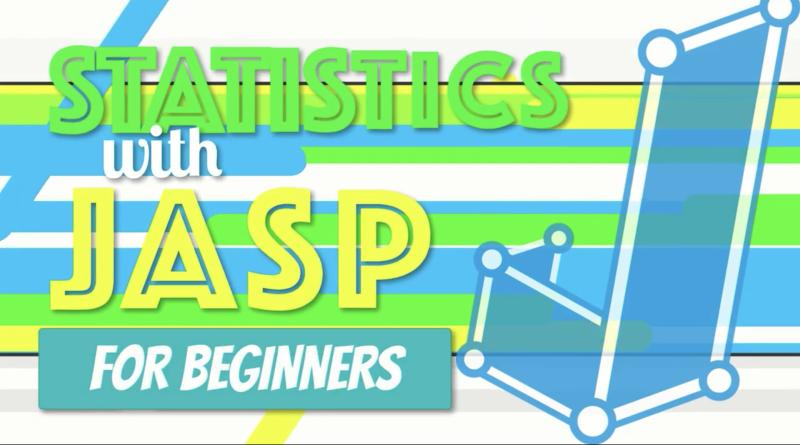# Guide/Tutorial## Video: Computing Central Tendency and Variability in JASP

Concise video (9:50) from Research by Design in which, “We walk through the steps to identify three measures of central tendency (mean, median, and mode) using JASP. This is an excellent introduction to JASP software and some of the functions that we will use throughout the introductory statistics course.” (7:41) “We will learn how to …## Video: Computing Frequencies and Creating an APA-Style Frequency Table Using JASP, Excel, and Word

Concise video from Research by Design in which, “We create an APA-style frequency table with scores in descending order, simple frequency, relative frequency, cumulative frequency, percentile, and sample size. We use JASP for the statistics, Excel for the formatting, and wrap it all up for presentation in Word. These same techniques work with open-source word …## Video: Central Limit Theorem and Distributions of Sample Means

This video (7:34) explains the central limit theorem, where distributions of means of samples come from, and how those distributions are normally distributed from StatQuest with Josh Starmer. Bam. Before watching, students should have a good understanding of the normal distribution. You can find the StatQuest website here.## Video: Statistical Significance What Are p Values? How Do We Interpret Them? How Do We Calculate Them?

A video (11:21) (which includes an “awesome song” in the beginning) that explains p values and their interpretation from StatQuest with Josh Starmer. Bam. Students should watch this before the video (below) on calculating p values. If you’re interested in how to calculate p values, this video (25:14) gives a thorough explanation. You can find …## Video: What is a Distribution? Normal Distributions, Clearly Explained

A short (5:14) video that introduces statistical distributions from StatQuest with Josh Starmer. This is great for students to watch before a discussion of the normal distribution. A short (5:12) video that clearly explains normal distributions from StatQuest with Josh Starmer. You can find the StatQuest website here.## Tutorial: Regression (WISE)

Online tutorial that guides students through a research study and data analysis using regression. There are 4 modules total, with the first three being most appropriate for an introductory course. Module 3 is short and could be used as an in-class demonstration. Module 4 is more advanced. During the tutorial students will start with a …## Tutorial: t-test for Independent Means/Samples (WISE)

Online tutorial that guides students through a research study and data analysis using a t-test for independent means/samples. This particular study compares differences in child rearing practices in Belize and Samoa. During the tutorial students will need to review data for data entry errors, look at descriptive statistics, form a hypothesis, perform the statistic, and …